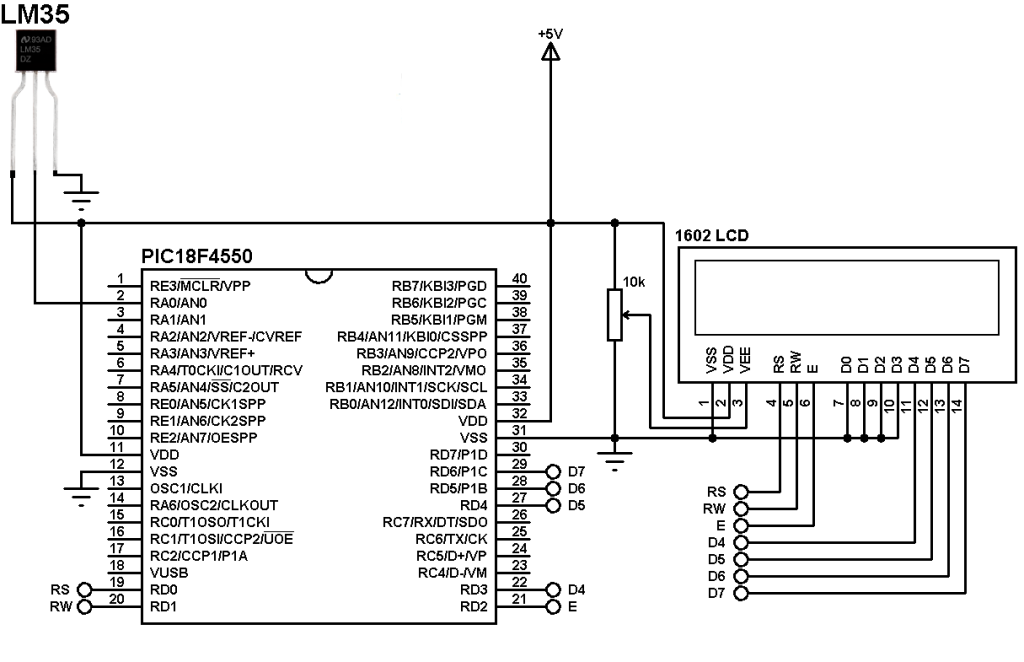# Interfacing LM35 temperature sensor with PIC18F4550 – CCS C

Interfacing PIC18F4550 with LM35

This small topic shows the circuit diagram and CCS C code of the interfacing of LM35 temperature sensor with PIC18F4550 microcontroller.
The LM35 temperature sensor is three pin device (VCC, OUT and GND) with an output voltage linearly related to Centigrade temperature. Since the LM35 output varies with dependent to the temperature we need ADC (Analog-to-Digital Converter) module to measure this voltage. The ADC module converts analog data into digital data.

The LM35 output has linear +10mV/°C scale factor means the following:
If the output voltage =   10mV —> temperature =   1°C
If the output voltage = 100mV —> temperature = 10°C
If the output voltage = 200mV —> temperature = 20°C
If the output voltage = 370mV —> temperature = 37°C
and so on.

LM35 Futures (from datasheet):

• Calibrated Directly in ° Celsius (Centigrade)
• Linear + 10 mV/°C Scale Factor
• 0.5°C Ensured Accuracy (at +25°C)
• Rated for Full −55°C to +150°C Range
• Suitable for Remote Applications
• Low Cost Due to Wafer-Level Trimming
• Operates from 4 to 30 V
• Less than 60-μA Current Drain
• Low Self-Heating, 0.08°C in Still Air
• Nonlinearity Only ±¼°C Typical
• Low Impedance Output, 0.1 Ω for 1 mA Load

Hardware Required:

• PIC18F4550 microcontroller
• LM35 temperature sensor  — datasheet
• 1602 LCD screen
• 10K ohm variable resistor
• 5V voltage source
• Jumper wires

Interfacing PIC18F4550 with LM35 sensor circuit:The output of the LM35 temperature sensor is connected to analog channel 0 (AN0) of the PIC18F4550 microcontroller.
In this example the MCU uses its internal oscillator and MCLR pin function is disabled.

Interfacing PIC18F4550 with LM35 temperature sensor C code:
The C code below was tested with CCS PIC C compiler version 5.051.

Reading voltage quantity using the ADC gives us a number between 0 and 1023 (10-bit resolution), 0V is represented by 0 and 5V is represented by 1023. Converting back the ADC digital value is easy and we can use the following equation for that conversion:
Multiplying the previous result by 100 (LM35 scale factor is 10mV/°C = 0.01V/°C) will gives the actual temperature:
1.Lee Tian Wei says: